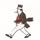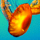## General Question# Care to try this 3D visualization problem while staying at home?

Asked by LostInParadise (26916) 2 weeks ago

First, the easy 2D version. Imagine a square with length a. At each corner, cut out a square of length b. Divide what remains into squares and rectangles.

Solution:
Join together the inner vertices of the cut out squares to create a square in the middle of length a – 2b. What remains will be 4 rectangles of lengths a and a – 2b.

Now start with a cube of length a, from which have been removed cubes of length b at the cube’s 8 vertices. Divide what remains into cubes and rectangular solids. I hope you will not find this too difficult, and that you will appreciate how nicely everything fits together.

Observing members: 0Composing members: 0“Divide what remains into cubes and rectangular solids”? Isn’t the number and variety of cubes and rectangular solids infinite?

stanleybmanly (21508)“Great Answer” (1) Flag as…Yes, but I am looking for one way of doing it, which I suspect is the minimum number of components.

This is what I had in mind. Analogously to the 2D case, join the innermost vertices of the cut out cubes to form a cube in the center. It will have length a – 2b. Let’s call this cube C.

Consider the top face of C. It has two sides of length a – 2b. Extend this face upward until it meets the original cube. This gives a rectangular solid with lengths a-2b, a – 2b and b. Repeat this for each of the remaining five faces of C. We end up with 6 rectangular solids with lengths a-2b, a-2b and b.

Now comes the difficult part of the visualization. Consider the edge between the top and left faces of C. We now have a region running along this edge between the solids we just constructed on the tops of these two faces. It is a rectangular solid of lengths a-2b, b and b. There are 12 of these solids, one for each edge of C, and we are now done.

One way of checking that our visualization is correct is to see if the sum of the volumes of the components, including the 8 cubes we originally removed, add up to the volume a cubed. This looks like a lot of work, but there is a way of simplifying the work. We note that a = (a-2b)+2b, so a cubed is the cube of the expression on the right. If you cube the right hand side, you get 4 expressions in terms of powers of (a-2b) and b. These coincide with the volumes of the 4 types of components of a, and the coefficients of 1, 6, 12 and 8 match the number of each type of component we used.

LostInParadise (26916)“Great Answer” (1) Flag as…The visualization isn’t that difficult once you appreciate that the large cube must contain 16 identical rectangular solids running the length of and abutting the axes of the big cube with their square faces bordering and congruent with the 8 corner cubes. Yet and still the toughest part of the “visualization” is expressing it in language. A picture is better than a thousand words.

stanleybmanly (21508)“Great Answer” (1) Flag as…Or is it 12 of the rectangular solids? I’m getting a headache.

stanleybmanly (21508)“Great Answer” (1) Flag as…I’m not that bored ;)

SEKA (4085)“Great Answer” (0) Flag as…or